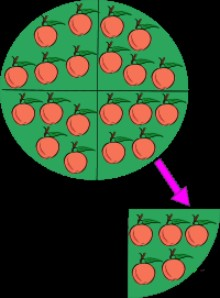Examples for business, study, careers, love, and more...
Examples :: Lesson Plans :: Example of Mathematics Lesson Plan:Dividing Three Digit Numbers by One

# Example of Mathematics Lesson Plan:Dividing Three Digit Numbers by One20 ÷4=5
1. Objective/s:

Divides 3- digit numbers by 1- digit numbers with out regrouping
2. Subject matter:
1. Dividing 3 digit numbers by 1-= digit numbers with out regrouping
2. Integration:
Character Education: Industriousness and thrift
Science: Animal hatched from egg

3. References/ Material/s:
1. Math handbook
2. Charts

4. Procedure:
1. Drill:
Give the division for each multiplication fact
Example: 3x5=15 15/3=5
Begin here:
4×9=36____/=_____=______
6×8=48____/=_____=______
7×6=42____/=_____=______
8×9=72____/=_____=______
9×6=54____/=_____=______
2. Presentation:
In a candy factory 680 chocolate bars were packed in 5 boxes. How many chocolate Bars were there in each box?
```
5÷680
5
18
15 (5x3)
30(5x6)
30
```
3. Analysis
4. Step 1:
Start at the hundreds place
Divide 6 by 5
Write 1 in the hundred places
Multiply 1 by 5=5
Subtract: 6 -5=1
Bring down 8

Step 2:
Divide 18 by 5=3
Multiply 3 by 5=15
Subtract 18-15=3

Step 3:
Bring down 0 beside 3
Divide 30 by 5=6
Multiply 6 by 5=30
Subtract 30-30=0
5. Practice Exercise
6. Find the quotient:

 2 ÷ 112 4 ÷ 452 3 ÷ 441 6 ÷ 270 6 ÷ 678
7. Application:
1. Fixing Skills:
Nino, a grade 3 pupil, helps his father sell balut at night. For 3 nights, was able to sell 105 pieces of balut. How many pieces of balut were sold each night?
2. Character Education Integration:
What kind of boy is Nino? In what other ways can children like you to help
Meet family expenses?
3. Science Integration:
How are young animals born? Name other animals hatched from egg.
5. Divide:
6.  3 ÷ 363 5 ÷ 5605 2 ÷ 426 4 ÷ 492 6 ÷ 678